$\newcommand{\NN}{\mathbb{N}} \newcommand{\CC}{\mathbb{C}} \newcommand{\GG}{\mathbb{G}} \newcommand{\LL}{\mathbb{L}} \newcommand{\PP}{\mathbb{P}} \newcommand{\QQ}{\mathbb{Q}} \newcommand{\RR}{\mathbb{R}} \newcommand{\VV}{\mathbb{V}} \newcommand{\ZZ}{\mathbb{Z}} \newcommand{\FF}{\mathbb{F}} \newcommand{\KK}{\mathbb{K}} \newcommand{\UU}{\mathbb{U}} \newcommand{\EE}{\mathbb{E}} \newcommand{\Aa}{\mathcal{A}} \newcommand{\Bb}{\mathcal{B}} \newcommand{\Cc}{\mathcal{C}} \newcommand{\Dd}{\mathcal{D}} \newcommand{\Ee}{\mathcal{E}} \newcommand{\Ff}{\mathcal{F}} \newcommand{\Gg}{\mathcal{G}} \newcommand{\Hh}{\mathcal{H}} \newcommand{\Ii}{\mathcal{I}} \newcommand{\Jj}{\mathcal{J}} \newcommand{\Kk}{\mathcal{K}} \newcommand{\Ll}{\mathcal{L}} \newcommand{\Mm}{\mathcal{M}} \newcommand{\Nn}{\mathcal{N}} \newcommand{\Oo}{\mathcal{O}} \newcommand{\Pp}{\mathcal{P}} \newcommand{\Qq}{\mathcal{Q}} \newcommand{\Rr}{\mathcal{R}} \newcommand{\Ss}{\mathcal{S}} \newcommand{\Tt}{\mathcal{T}} \newcommand{\Uu}{\mathcal{U}} \newcommand{\Vv}{\mathcal{V}} \newcommand{\Ww}{\mathcal{W}} \newcommand{\Xx}{\mathcal{X}} \newcommand{\Yy}{\mathcal{Y}} \newcommand{\Zz}{\mathcal{Z}} \newcommand{\al}{\alpha} \newcommand{\la}{\lambda} \newcommand{\ga}{\gamma} \newcommand{\Ga}{\Gamma} \newcommand{\La}{\Lambda} \newcommand{\Si}{\Sigma} \newcommand{\si}{\sigma} \newcommand{\be}{\beta} \newcommand{\de}{\delta} \newcommand{\De}{\Delta} \renewcommand{\phi}{\varphi} \renewcommand{\th}{\theta} \newcommand{\om}{\omega} \newcommand{\Om}{\Omega} \renewcommand{\epsilon}{\varepsilon} \newcommand{\Calpha}{\mathrm{C}^\al} \newcommand{\Cbeta}{\mathrm{C}^\be} \newcommand{\Cal}{\text{C}^\al} \newcommand{\Cdeux}{\text{C}^{2}} \newcommand{\Cun}{\text{C}^{1}} \newcommand{\Calt}{\text{C}^{#1}} \newcommand{\lun}{\ell^1} \newcommand{\ldeux}{\ell^2} \newcommand{\linf}{\ell^\infty} \newcommand{\ldeuxj}{{\ldeux_j}} \newcommand{\Lun}{\text{\upshape L}^1} \newcommand{\Ldeux}{\text{\upshape L}^2} \newcommand{\Lp}{\text{\upshape L}^p} \newcommand{\Lq}{\text{\upshape L}^q} \newcommand{\Linf}{\text{\upshape L}^\infty} \newcommand{\lzero}{\ell^0} \newcommand{\lp}{\ell^p} \renewcommand{\d}{\ins{d}} \newcommand{\Grad}{\text{Grad}} \newcommand{\grad}{\text{grad}} \renewcommand{\div}{\text{div}} \newcommand{\diag}{\text{diag}} \newcommand{\pd}{ \frac{ \partial #1}{\partial #2} } \newcommand{\pdd}{ \frac{ \partial^2 #1}{\partial #2^2} } \newcommand{\dotp}{\langle #1,\,#2\rangle} \newcommand{\norm}{|\!| #1 |\!|} \newcommand{\normi}{\norm{#1}_{\infty}} \newcommand{\normu}{\norm{#1}_{1}} \newcommand{\normz}{\norm{#1}_{0}} \newcommand{\abs}{\vert #1 \vert} \newcommand{\argmin}{\text{argmin}} \newcommand{\argmax}{\text{argmax}} \newcommand{\uargmin}{\underset{#1}{\argmin}\;} \newcommand{\uargmax}{\underset{#1}{\argmax}\;} \newcommand{\umin}{\underset{#1}{\min}\;} \newcommand{\umax}{\underset{#1}{\max}\;} \newcommand{\pa}{\left( #1 \right)} \newcommand{\choice}{ \left\{ \begin{array}{l} #1 \end{array} \right. } \newcommand{\enscond}{ \left\{ #1 \;:\; #2 \right\} } \newcommand{\qandq}{ \quad \text{and} \quad } \newcommand{\qqandqq}{ \qquad \text{and} \qquad } \newcommand{\qifq}{ \quad \text{if} \quad } \newcommand{\qqifqq}{ \qquad \text{if} \qquad } \newcommand{\qwhereq}{ \quad \text{where} \quad } \newcommand{\qqwhereqq}{ \qquad \text{where} \qquad } \newcommand{\qwithq}{ \quad \text{with} \quad } \newcommand{\qqwithqq}{ \qquad \text{with} \qquad } \newcommand{\qforq}{ \quad \text{for} \quad } \newcommand{\qqforqq}{ \qquad \text{for} \qquad } \newcommand{\qqsinceqq}{ \qquad \text{since} \qquad } \newcommand{\qsinceq}{ \quad \text{since} \quad } \newcommand{\qarrq}{\quad\Longrightarrow\quad} \newcommand{\qqarrqq}{\quad\Longrightarrow\quad} \newcommand{\qiffq}{\quad\Longleftrightarrow\quad} \newcommand{\qqiffqq}{\qquad\Longleftrightarrow\qquad} \newcommand{\qsubjq}{ \quad \text{subject to} \quad } \newcommand{\qqsubjqq}{ \qquad \text{subject to} \qquad }$

Geodesic Distance with Poisson Equation

# Geodesic Distance with Poisson Equation

This tour explores the method detailed in the paper [CraneWeischedelWardetzky13] to compute geodesic distance by simply solving a Poisson equation.

## Installing toolboxes and setting up the path.

You need to download the following files: signal toolbox, general toolbox and graph toolbox.

You need to unzip these toolboxes in your working directory, so that you have toolbox_signal, toolbox_general and toolbox_graph in your directory.

For Scilab user: you must replace the Matlab comment '%' by its Scilab counterpart '//'.

Recommandation: You should create a text file named for instance numericaltour.sce (in Scilab) or numericaltour.m (in Matlab) to write all the Scilab/Matlab command you want to execute. Then, simply run exec('numericaltour.sce'); (in Scilab) or numericaltour; (in Matlab) to run the commands.

Execute this line only if you are using Matlab.

getd = @(p)path(p,path); % scilab users must *not* execute this


Then you can add the toolboxes to the path.

getd('toolbox_signal/');
getd('toolbox_general/');
getd('toolbox_graph/');

Warning: Name is nonexistent or not a directory: toolbox_signal
Warning: Name is nonexistent or not a directory: toolbox_general
Warning: Name is nonexistent or not a directory: toolbox_graph


## Gradient, Divergence and Laplacian on Surfaces

The topology of a triangulation is defined via a set of indexes $$\Vv = \{1,\ldots,n\}$$ that indexes the $$n$$ vertices, a set of edges $$\Ee \subset \Vv \times \Vv$$ and a set of $$m$$ faces $$\Ff \subset \Vv \times \Vv \times \Vv$$.

We load a mesh. The set of faces $$\Ff$$ is stored in a matrix $$F \in \{1,\ldots,n\}^{3 \times m}$$. The positions $$x_i \in \RR^3$$, for $$i \in V$$, of the $$n$$ vertices are stored in a matrix $$X_0 = (x_{0,i})_{i=1}^n \in \RR^{3 \times n}$$.

clear options;
name = 'elephant-50kv';
options.name = name; % useful for displaying


Number $$n$$ of vertices and number $$m$$ of faces.

n = size(X,2);
m = size(F,2);


Display the mesh in 3-D.

options.lighting = 1;
clf;
plot_mesh(X,F,options);
axis('tight');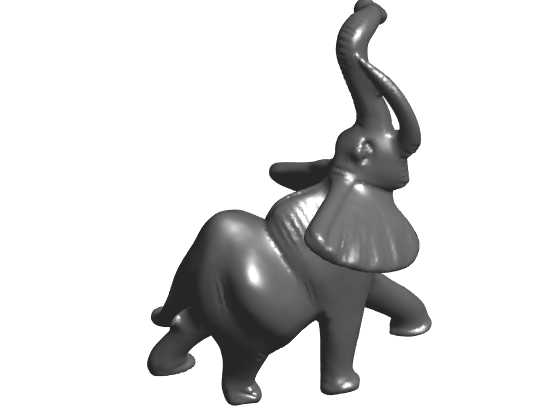In this tour, we use piecewise linear finite element to compute the gradient operators, which in turns allows us to compute the divergence (its transposed operator) and the Laplacian (as the composition of the divergence and the gradient).

The gradient operator $$\nabla$$ can be understood as a collection of 3 sparse matrices $$(\nabla_s)_{s=1,2,3}$$ of size $$(m,n)$$ that computes each coordinate of $$\nabla u=(\nabla_s u)_{s=1,2,3}$$ through the formula, for each face $$f$$, $(\nabla u)_f = \frac{1}{2A_f} \sum_{i \in f} u_i (N_f \wedge e_i)$ where $$A_f$$ is the area of face $$f$$, $$N_f$$ is the normal to the face, $$e_i$$ is the edge opposite to vertex $$i$$, and $$\wedge$$ is the cross product in $$\RR^3$$.

Callback to get the coordinates of all the vertex of index $$i=1,2,3$$ in all faces.

XF = @(i)X(:,F(i,:));


Compute un-normalized normal through the formula $$e_1 \wedge e_2$$ where $$e_i$$ are the edges.

Na = cross( XF(2)-XF(1), XF(3)-XF(1) );


Compute the area of each face as half the norm of the cross product.

amplitude = @(X)sqrt( sum( X.^2 ) );
A = amplitude(Na)/2;


Compute the set of unit-norm normals to each face.

normalize = @(X)X ./ repmat(amplitude(X), [3 1]);
N = normalize(Na);


Populate the sparse entries of the matrices for the operator implementing $$\sum_{i \in f} u_i (N_f \wedge e_i)$$.

I = []; J = []; V = []; % indexes to build the sparse matrices
for i=1:3
% opposite edge e_i indexes
s = mod(i,3)+1;
t = mod(i+1,3)+1;
% vector N_f^e_i
wi = cross(XF(t)-XF(s),N);
% update the index listing
I = [I, 1:m];
J = [J, F(i,:)];
V = [V, wi];
end


Sparse matrix with entries $$1/(2A_f)$$.

dA = spdiags(1./(2*A(:)),0,m,m);


GradMat = {};
for k=1:3
end


$$\nabla$$ gradient operator.

Grad = @(u)[GradMat{1}*u, GradMat{2}*u, GradMat{3}*u]';


Compute divergence matrices as transposed of grad for the face area inner product.

dAf = spdiags(2*A(:),0,m,m);


Div operator.

Div = @(q)DivMat{1}*q(1,:)' + DivMat{2}*q(2,:)' + DivMat{3}*q(3,:)';


Laplacian operator as the composition of grad and div.

Delta = DivMat{1}*GradMat{1} + DivMat{2}*GradMat{2} + DivMat{3}*GradMat{3};


Cotan of an angle between two vectors.

cota = @(a,b)cot( acos( dot(normalize(a),normalize(b)) ) );


Compute cotan weights Laplacian.

I = []; J = []; V = []; % indexes to build the sparse matrices
Ia = []; Va = []; % area of vertices
for i=1:3
% opposite edge e_i indexes
s = mod(i,3)+1;
t = mod(i+1,3)+1;
ctheta = cota(XF(s)-XF(i), XF(t)-XF(i));
% ctheta = max(ctheta, 1e-2); % avoid degeneracy
% update the index listing
I = [I, F(s,:), F(t,:)];
J = [J, F(t,:), F(s,:)];
V = [V, ctheta, ctheta];
% update the diagonal with area of face around vertices
Ia = [Ia, F(i,:)];
Va = [Va, A];
end
Ac = sparse(Ia,Ia,Va,n,n);
% Cotan weights
Wc = sparse(I,J,V,n,n);
% Laplacian with cotan weights.
DeltaCot = spdiags(full(sum(Wc))', 0, n,n) - Wc;


Check that the Laplacian with cotan weights is actually equal to the composition of divergence and gradient.

fprintf('Should be 0: %e\n', norm(Delta-DeltaCot, 'fro')/norm(Delta, 'fro'));

Should be 0: 1.604879e-10


Display a function $$f$$ on the mesh.

f = X(2,:);
options.face_vertex_color = f(:);
clf; plot_mesh(X,F,options);
axis('tight');
colormap parula(256);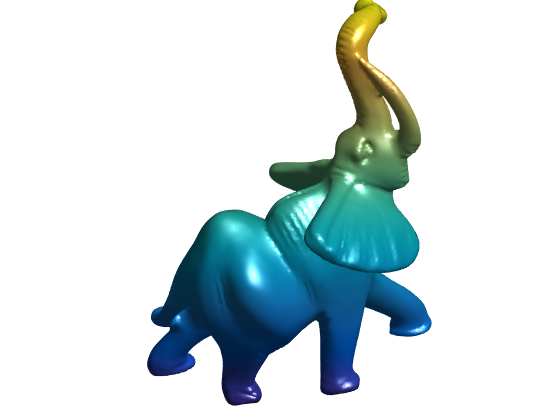Display its Laplacian.

g = Delta*f(:);
g = clamp(g, -3*std(g), 3*std(g));
options.face_vertex_color = rescale(g);
clf; plot_mesh(X,F,options);
axis('tight');
colormap parula(256);## Heat Diffusion and Time Stepping

The method developped in [CraneWeischedelWardetzky13] relies on the fact that the level set of the geodesic distance function to a starting point $$i$$ agrees with the level set of the solution of the heat diffusion when the time of diffusion tends to zero. This fundamental result is proved in [Varadhan67].

In fact, the same result holds true when replacing the heat diffusion solution by a single Euler implicit step in time, with time step $$t$$. This means one should consider the solution $$u$$ to the equation $(\text{Id}+t \Delta) u = \delta_i$ where $$\delta_i$$ is the Dirac vector at vertex index $$i$$.

Select index $$i$$.

i = 21000;


Set time $$t$$.

t = 1000;


Solve the linear system.

delta = zeros(n,1);
delta(i) = 1;
u = (Ac+t*Delta)\delta;


Display this solution.

options.face_vertex_color = u;
clf; plot_mesh(X,F,options);
axis('tight');
colormap parula(256);Exercice 1: (check the solution) Solve the heat diffusion equation $\frac{\partial g}{\partial t} = -\Delta g$ by performing several implicit time stepping.

exo1;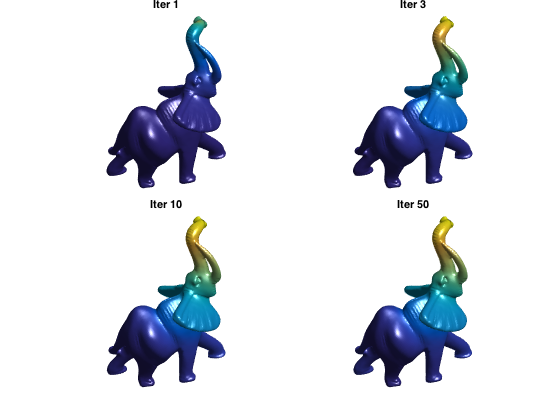## Geodesic in Heat Method

The main point of the method [CraneWeischedelWardetzky13] is to retrieve an approximation of the distance function $$\phi$$ from the level set of implicit heat diffusion step $$u$$.

This is achieved by using the fact that $$\norm{\nabla \phi}=1$$, i.e. one should have $\nabla \phi \approx -\frac{\nabla u}{\norm{\nabla u}}.$ Solving this equation in the least square sense leads to the resolution of a Poisson equation.

Compute the solution $$u$$ with explicit time stepping.

t = .1;
u = (Ac+t*DeltaCot)\delta;


g = Grad(u);


Normalize it to obtain $h = -\frac{\nabla u}{\norm{\nabla u}}.$

h = -normalize(g);


Integrate it back by solving $\Delta \phi = \text{div}(h).$

phi = Delta \ Div(h);


Display.

options.face_vertex_color = phi;
clf; plot_mesh(X,F,options);
axis('tight');
colormap parula(256);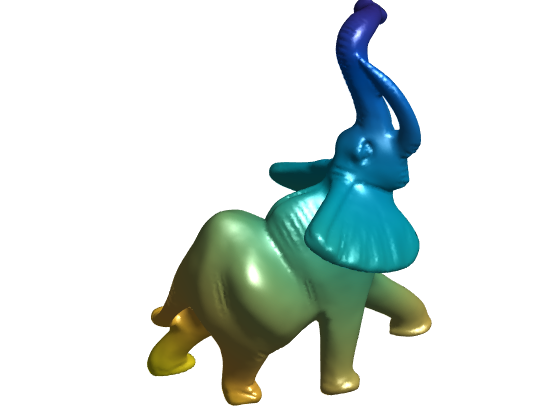Functions for displaying the level sets of the distance.

p = 30;
DispFunc = @(phi)cos(2*pi*p*phi);


Same, but using a different colormap.

options.face_vertex_color = DispFunc(phi);
clf; plot_mesh(X,F,options);
axis('tight');
colormap parula(256);Exercice 2: (check the solution) Display the result obtained for several time $$t$$.

exo2;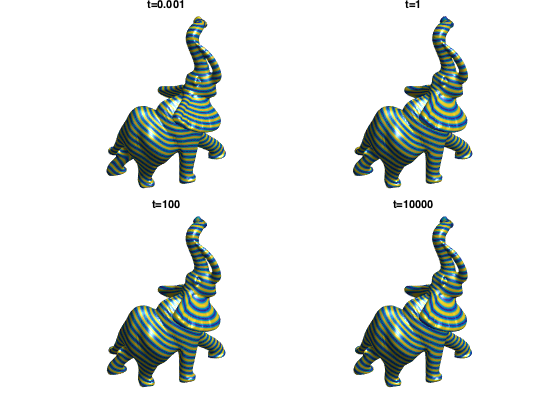Exercice 3: (check the solution) Compute distances from an increasing number of starting points that are computed using a farthest point sampling.

exo3;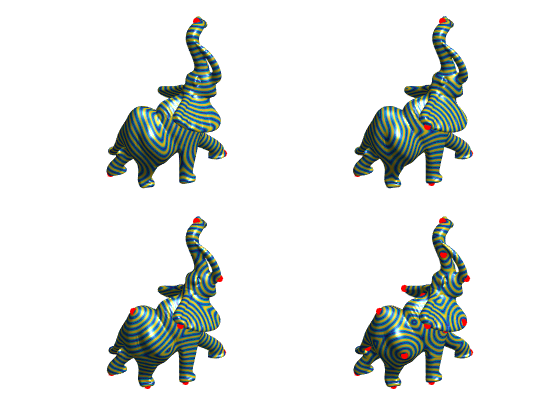## Bibliography

• [CraneWeischedelWardetzky13] K. Crane, C. Weischedel, M. Wardetzky, Geodesics in heat: A new approach to computing distance based on heat flow, ACM Transactions on Graphics , vol. 32, no. 5, pp. 152:1-152:11, 2013.
• [Varadhan67] S.R.S. Varadhan, On the behavior of the fundamental solution of the heat equation with variable coefficients Communications on Pure and Applied Mathematics 20, 2, 431-455, 1967